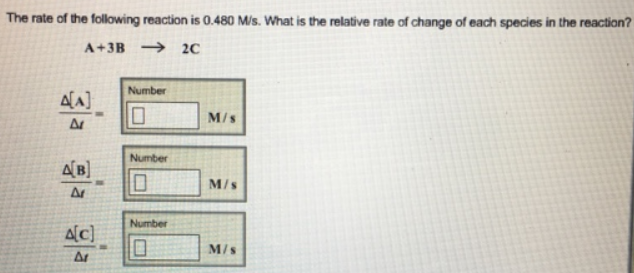# Problem: The rate of the following reaction is 0.480 M/s. What is the relative rat of change of each species in the reaction? A + 3B → 2C Δ[A]/Δt = Δ[B]/Δt = Δ[C]/Δt =

###### FREE Expert Solution
82% (164 ratings)###### Problem Details

The rate of the following reaction is 0.480 M/s. What is the relative rat of change of each species in the reaction?

A + 3B → 2C

Δ[A]/Δt =

Δ[B]/Δt =

Δ[C]/Δt =